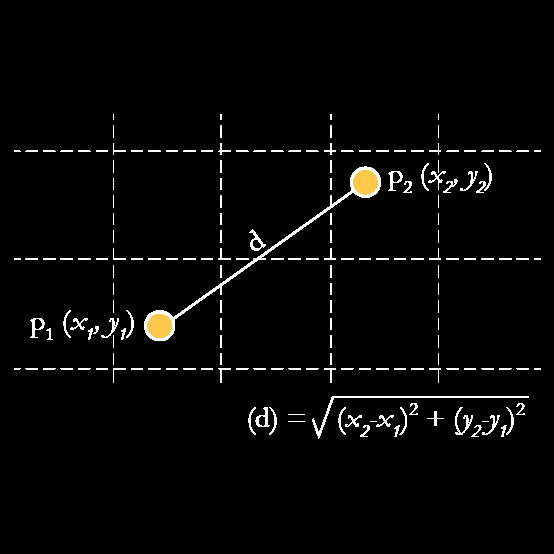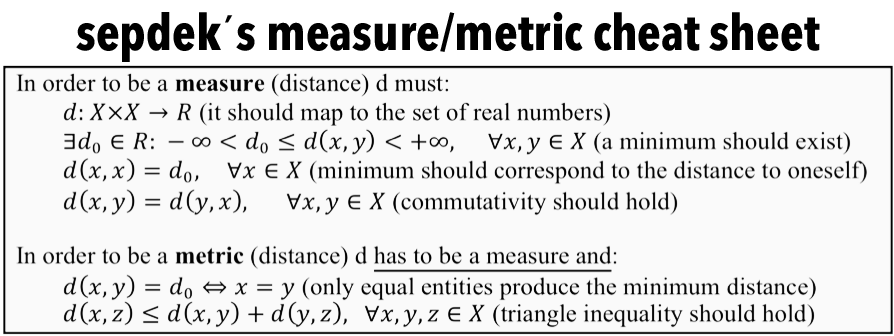#### Measure and Metric cheat sheet

October 8, 2016In order to be a measure (distance) d must:$\displaystyle d:X \times X \rightarrow R \; \text{(it should map to the set of real numbers)}$$\displaystyle \exists d_0 \in R: -\infty \textless d_0 \leq d(x,y) \textless +\infty , \; \forall x,y \in X \; \text{(a minimum should exist)}$$\displaystyle d(x,x)=d_0, \forall x \in X \; \text{(minimum should correspond to the distance to oneself)}$$\displaystyle d(x,y)=d(y,x), \; \forall x,y \in X \; \text{(commutativity should hold)}$

In order to be a metric (distance) d has to be a measure and:$\displaystyle d(x,y)=d_0 \Leftrightarrow x=y \; \text{(only equal entities produce the minimum distance)}$$\displaystyle d(x,z) \leq d(x,y)+d(y,z), \; \forall x,y,z \in X \; \text{(triangle inequality should hold)}$

…and here is the cheat sheet: